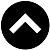Type something and hit enter

## PagesINPUTS:
1) Dividend Yield:
The latest dividend for the last 12 months are 3 cents and 2 cents, Dividend Yield is 5/169 = 3%. However, there are 2 times of 1:100 treasury distribution, equivalent to 2%, so I input Dividend Yield of 3%+2% = 5%.
Note: Higher dividend yield will make the fair value lower.

2) Interest Rate (i.e. Return if to buy mother share):
Make reference to i3investor.com, the following are the target prices given by investment banks:
a) AffinHwang: 2.05
b) Aminvest: 1.98
c) HLG: 2.17
d) Kenanga: 1.60
e) MIDF: 1.55
Take average, (2.05+1.98+2.17+1.60+1.55) / 5 = 1.87, the room = 1.87 / 1.69 - 1 = 10.65%
Note: Target prices is understood as 12 months target net of dividend. Therefore the expected return for people who buy mother share will be 5%+10.65% = 15.65%. However, I normally limit it to 8% for input to the calculator.

3) Volatility:
Calculate from the last 90 trading days, the Volatility of the mother share is 17.70%.

4) Underlying:
Mother share at yesterday close was 1.69.

5) Exercise Price:
The exercise price is quite rare and special for SUNWAY-WB, it is stepping down 7 cents every year, the exercise prices for the 7 years life span of SUNWAY-WB are 1.86,1.79, 1.72, 1.65, 1.58, 1.51 and 1.44 respectively. Of course people will wait until the final year only then to convert SUNWAY-WB to mother share. So the input to calculator should be 1.44.

6) Valuation Date:
Today.

7) Exercise Date (i.e. Expiry):
The actual expiry is on 3rd October 2024. However, make reference to records and we can expect there will be dividend ex-dated in the month of September, it is better to convert SUNWAY-WB before that ex-date to enjoy the dividend. So for calculation purpose we should input expiry date earlier, say 3 months earlier on 3rd july 2024.

OUTPUTS:
8) The fair value for SUNWAY-WB is 40.2 cents, very much cheaper than the market price of 34.5 cents, or 34.5 / 40.2 = 14% discount.